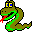#Boost C++ Libraries

...one of the most highly regarded and expertly designed C++ library projects in the world.#minimum_degree_ordering

```  template <class AdjacencyGraph, class OutDegreeMap,
class InversePermutationMap,
class PermutationMap, class SuperNodeSizeMap, class VertexIndexMap>
void minimum_degree_ordering
OutDegreeMap outdegree,
InversePermutationMap inverse_perm,
PermutationMap perm,
SuperNodeSizeMap supernode_size, int delta, VertexIndexMap id)
```

The minimum degree ordering algorithm [21, 35] is a fill-in reduction matrix reordering algorithm. When solving a system of equations such as A x = b using a sparse version of Cholesky factorization (which is a variant of Gaussian elimination for symmetric matrices), often times elements in the matrix that were once zero become non-zero due to the elimination process. This is what is referred to as "fill-in", and fill-in is bad because it makes the matrix less sparse and therefore consume more time and space in later stages of the elimintation and in computations that use the resulting factorization. Now it turns out that reordering the rows of a matrix can have a dramatic affect on the amount of fill-in that occurs. So instead of solving A x = b, we instead solve the reordered (but equivalent) system (P A PT)(P x) = P b. Finding the optimal ordering is an NP-complete problem (e.i., it can not be solved in a reasonable amount of time) so instead we just find an ordering that is "good enough" using heuristics. The minimum degree ordering algorithm is one such approach.

A symmetric matrix A is typically represented with an undirected graph, however for this function we require the input to be a directed graph. Each nonzero matrix entry A(i, j) must be represented by two directed edges (e(i,j) and e(j,i)) in G.

The output of the algorithm are the vertices in the new ordering.

```  inverse_perm[new_index[u]] == old_index[u]
```

and the permutation from the old index to the new index.

```  perm[old_index[u]] == new_index[u]
```

The following equations are always held.

```  for (size_type i = 0; i != inverse_perm.size(); ++i)
perm[inverse_perm[i]] == i;
```

### Parameters

A directed graph. The graph's type must be a model of Adjacency Graph, Vertex List Graph, Incidence Graph, and Mutable Graph. In addition, the functions num_vertices() and out_degree() are required.
• OutDegreeMap outdegree  (WORK)
This is used internally to store the out degree of vertices. This must be a LvaluePropertyMap with key type the same as the vertex descriptor type of the graph, and with a value type that is an integer type.
• InversePermutationMap inverse_perm  (OUT)
The new vertex ordering, given as the mapping from the new indices to the old indices (an inverse permutation). This must be an LvaluePropertyMap with a value type and key type a signed integer.
• PermutationMap perm  (OUT)
The new vertex ordering, given as the mapping from the old indices to the new indices (a permutation). This must be an LvaluePropertyMap with a value type and key type a signed integer.
• SuperNodeSizeMap supernode_size  (WORK/OUT)
This is used internally to record the size of supernodes and is also useful information to have. This is a LvaluePropertyMap with an unsigned integer value type and key type of vertex descriptor.
• int delta  (IN)
Multiple elimination control variable. If it is larger than or equal to zero then multiple elimination is enabled. The value of delta specifies the difference between the minimum degree and the degree of vertices that are to be eliminated.
• VertexIndexMap id  (IN)
Used internally to map vertices to their indices. This must be a Readable Property Map with key type the same as the vertex descriptor of the graph and a value type that is some unsigned integer type.

### Example

See example/minimum_degree_ordering.cpp.

### Implementation Notes

UNDER CONSTRUCTION

It chooses the vertex with minimum degree in the graph at each step of simulating Gaussian elimination process.

This implementation provided a number of enhancements including mass elimination, incomplete degree update, multiple elimination, and external degree. See  for a historical survey of the minimum degree algorithm.

An elimination graph Gv is obtained from the graph G by removing vertex v and all of its incident edges and by then adding edges to make all of the vertices adjacent to v into a clique (that is, add an edge between each pair of adjacent vertices if an edge doesn't already exist). Suppose that graph G is the graph representing the nonzero structure of a matrix A. Then performing a step of Guassian elimination on row i of matrix A is equivalent to

 Copyright © 2001 Lie-Quan Lee, Indiana University (llee@cs.indiana.edu) Jeremy Siek, Indiana University (jsiek@osl.iu.edu)